# How to drop a level from a multi-level column index in Pandas Dataframe ?

• Last Updated : 21 Apr, 2021

In this article, we will learn about how to drop a level from a multi-level column index. But before that, we need to know what is a multi-level index. A multi-level index dataframe is a type of dataframe that contains multiple level or hierarchical indexing.
In this article, we will be creating a dataframe of our own choice with multiple column indexing, and then we are going to drop off a level of our hierarchical indexing.

### Step by Step Implementation

Let’s understand this using step-by-step implementation with the help of an example.

Step 1: Import all the libraries required.

## Python3

 `# importing all important libraries``import` `pandas as pd`

Step 2: Create a multi-level column index Pandas Dataframe and show it.

We are creating a multi-index column using MultiIndex.from_tuples() which helps us to create multiple indexes one below another, and it is created column-wise.  After that, using pd.Dataframe() we are creating data and converting it into the tabular format with the column names as the multi-level indexes. Also, we are changing the index name of the table using df.index.

## Python3

 `# Creating a multilevel index ``index ``=` `pd.MultiIndex.from_tuples([(``"Group 1"``, ``"Group 1"``), ``                                   ``(``"Group 1"``, ``"Group 2"``),``                                   ``(``"Group 3"``,``"Group 3"``)])`` ` `# Creating a pandas dataframe with ``# multilevel-column indexing``df ``=` `pd.DataFrame([[``"Ross"``,``"Joey"``,``"Chandler"``],``                   ``[``"Rachel"``,"``","``Monica"]], ``                  ``columns``=``index)`` ` `# Labelling the dataframe index.``index ``=` `df. index``index. name ``=` `"F.R.I.E.N.D.S"`` ` `# Showing the above multi-index column``# dataframe``print``(df)`

Output: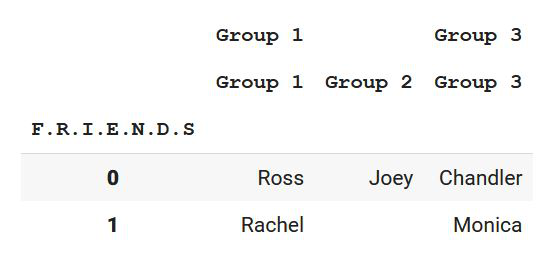Step 3: Drop the level(s) of the dataframe

Now a multi-level column index dataframe is created using python. Now let us implement the above concept now. We need to drop a level. We can do that using df.columns.droplevel(level=0). This helps us to drop an index level from the top that is of index 0.

## Python3

 `# Dropping a level down``df.columns ``=` `df.columns.droplevel(``0``)`

Step 4: Show the required result

## Python3

 `print``(df)`

Output: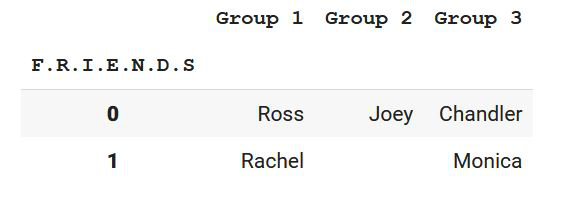Hence, we have been able to drop a level of index column successfully.

Let’s see some more examples based on the above approach.

Example 1:

In the next example, we will be dropping a level from a specific index in the multi-level column index. This can be done using the same syntax we have used earlier[df.columns.droplevel(level=0)] where if we specify the level number, then the following index gets deleted according to zero-based indexing. So let us move to the implementation of the concept.

## Python3

 `# importing all important libraries``import` `pandas as pd`` ` `# Creating a multilevel index ``index ``=` `pd.MultiIndex.from_tuples([(``"Company A"``, ``"Company B"``,``"Company C"``), ``                                   ``(``"Company A"``, ``"Company A"``,``"Company B"``),``                                   ``(``"Company A"``,``"Company B"``,``"Company C"``)])`` ` `# Creating a pandas dataframe with ``# multilevel-column indexing``df ``=` `pd.DataFrame([[``"Atreyi"``,``"Digangana"``,``"Sohom"``],``                   ``[``"Sujit"``,``"Bjon"``,``"Rajshekhar"``],``                   ``[``"Debosmita"``,``"Shatabdi"``,""]], ``                  ``columns``=``index)`` ` `# Labelling the dataframe index.``index ``=` `df. index``index. name ``=` `"ECE Placement"`` ` `# Showing the above multi-index column``# dataframe``print``(df)`

Output: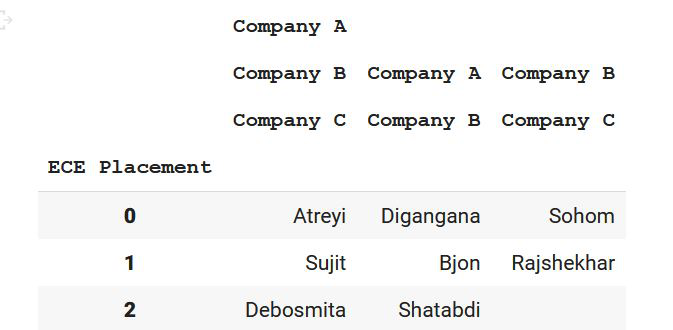Now, if we want to drop level with index 2, then let’s see what happens!

## Python3

 `# Dropping a level number 2``df.columns ``=` `df.columns.droplevel(``2``)``print``(df)`

Output: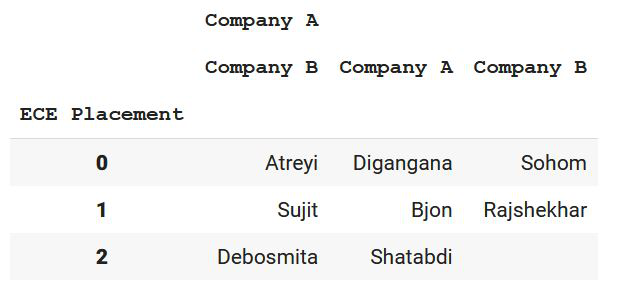Hence, we can observe that in the multi-level column index, we have successfully removed the level with index number 2.

Example 2:

In this example, we will be implementing more concepts of the multi-level index. We will be deleting multiple levels at the same time.

## Python3

 `# importing all important libraries``import` `pandas as pd`` ` `# Creating a multilevel index``index ``=` `pd.MultiIndex.from_tuples([(``"Company A"``, ``"Company B"``, ``"Company C"``),``                                   ``(``"Company A"``, ``"Company A"``, ``"Company B"``),``                                   ``(``"Company A"``, ``"Company B"``, ``"Company C"``)])`` ` `# Creating a pandas dataframe with``# multilevel-column indexing``df ``=` `pd.DataFrame([[``"Atreyi"``, ``"Digangana"``, ``"Sohom"``],``                   ``[``"Sujit"``, ``"Bjon"``, ``"Rajshekhar"``],``                   ``[``"Debosmita"``, ``"Shatabdi"``, ""]],``                  ``columns``=``index)`` ` `# Labelling the dataframe index.``index ``=` `df. index``index. name ``=` `"ECE Placement"`` ` `# Showing the above multi-index column``# dataframe``print``(df)`

Output:As we can see, every list of arrays contains the indexes column-wise. So, three arrays mean three columns and the number of values in the array refers to the number of rows. Let us delete multiple indexes from the dataframe now. We can do that using df.columns.droplevel(level=0) by calling it multiple times. But here is a catch!

## Python3

 `# Dropping a level down``df.columns ``=` `df.columns.droplevel(``0``)`` ` `# Dropping another level down``df.columns ``=` `df.columns.droplevel(``0``)`` ` `# Showing the dataframe``print``(df)`

As we can see, there are two droplevel statements with the level as 0. This is because, after the removal of a single level, the remaining ones get rearranged. So the level that was at index 1 will now come to index 0, Hence multiple droplevels are written in that case.

Output: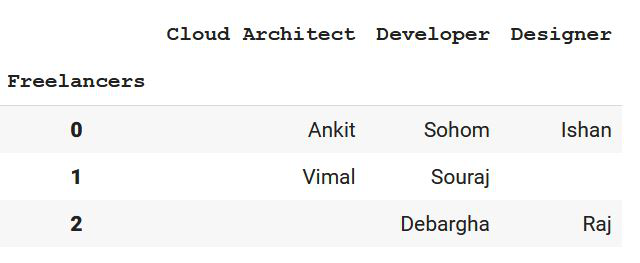Hence, level 0 and level 1 get removed, and we are left with only level 2 which is now shown as level 0.

Example 3:

In the last example, let us remove multiple levels from various positions in the dataframe.

## Python3

 `# importing all important libraries``import` `pandas as pd`` ` `# Creating a pandas dataframe``df ``=` `pd.DataFrame([[``"Coding"``, ``"System Design"``],``                   ``[``"DBMS"``, ``"Aptitude"``],``                   ``[``"Logical Reasoning"``, ``"Development"``]])`` ` `# Creating multilevel index from tuples``df.columns ``=` `pd.MultiIndex.from_tuples([(``'Group 1'``, ``'Group 2'``, ``'Group 3'``, ``'Group 4'``),``                                        ``(``'Group 3'``, ``'Group 4'``, ``'Group 5'``, ``'Group 6'``)],``                                       ``names``=``[``'level 1'``, ``'level 2'``, ``'level 3'``, ``'level 4'``])``# Showing the dataframe``print``(df)`

Output: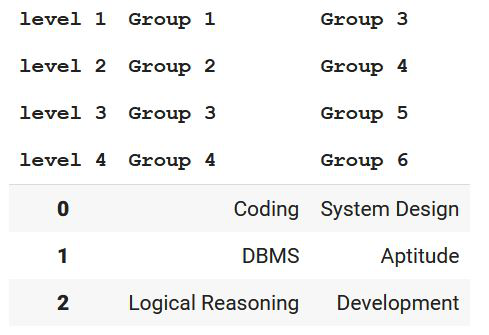Now let us remove level 1 and 3 respectively:

## Python3

 `# Dropping a level down(Level 1)``df.columns ``=` `df.columns.droplevel(``0``)`` ` `# Dropping a level down after``# re-arrangement(Level 2)``df.columns ``=` `df.columns.droplevel(``1``)`` ` `# Showing the dataframe``print``(df)`

As we can see, we have dropped a level down from index 0 in the first case. After re-arrangement level 2 will now come to the 0 indexes of the multi-level index dataframe. Now in order to remove level 3 now, we have to specify the level as 1 according to the 0-based indexing after re-arrangement. Now levels 2 and 4 will be shown in the resultant output.

Output: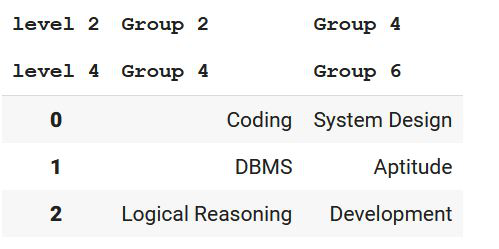My Personal Notes arrow_drop_up